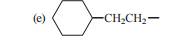Problem: Using the method outlined in Section 2.13, give an IUPAC name for each of the following alkyl groups, and classify each one as primary, secondary, or tertiary:

FREE Expert Solution
80% (432 ratings)
Problem Details

Using the method outlined in Section 2.13, give an IUPAC name for each of the following alkyl groups, and classify each one as primary, secondary, or tertiary:What scientific concept do you need to know in order to solve this problem?

Our tutors have indicated that to solve this problem you will need to apply the IUPAC Naming concept. You can view video lessons to learn IUPAC Naming Or if you need more IUPAC Naming practice, you can also practice IUPAC Naming practice problems .

What is the difficulty of this problem?

Our tutors rated the difficulty of Using the method outlined in Section 2.13, give an IUPAC nam... as low difficulty.

How long does this problem take to solve?

Our expert Organic tutor, Chris took 3 minutes to solve this problem. You can follow their steps in the video explanation above.

What textbook is this problem found in?

Our data indicates that this problem or a close variation was asked in . You can also practice practice problems .